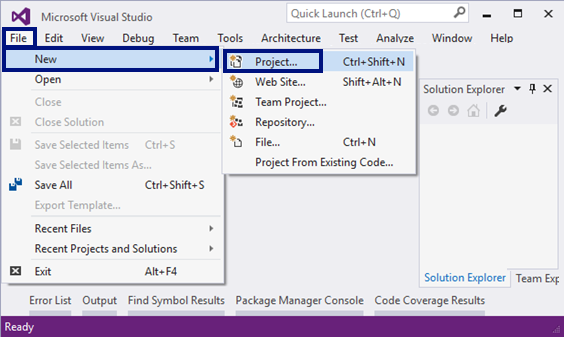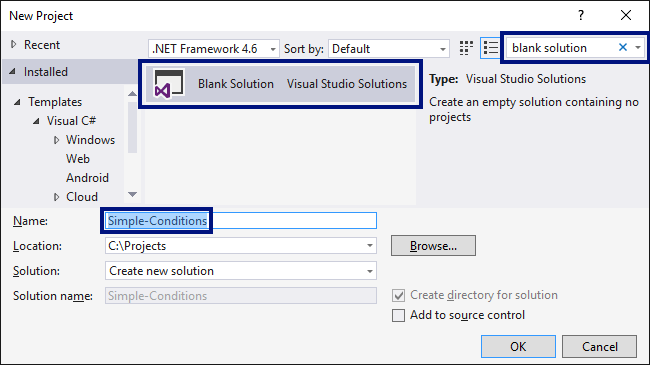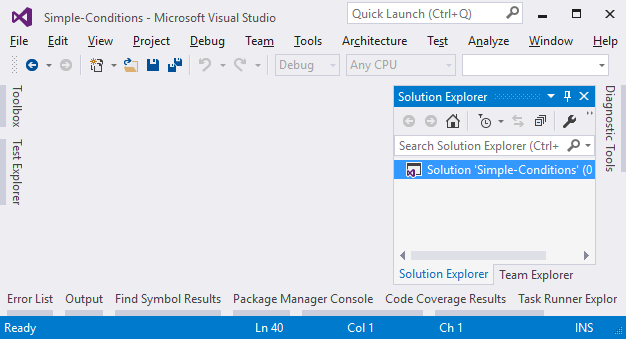# Exercises: Simple Conditions

Now let's practice the lessons learned in this chapter about of conditional statements if and if-else. We will solve a few practical exercises.

## Video: Chapter Summary

Watch the following video to summarize what we learned in this chapter: https://youtu.be/mdv28HD5qes.

## What We Learned in This Chapter?

Let's summarize what we learned in this chapter:

• Numbers can be compared by the ==, <, >, <=, >= and != operators:
Console.WriteLine(5 <= 10);  // True

• Simple if-conditions check a condition and execute a code block if it is true:
if (a > 5)
{
Console.WriteLine("The number a is bigger than 5");
}

• The if-else construction executes one of two blocks depending on whether a condition is true or false:
if (a > 5)
{
Console.WriteLine("The number a is bigger than 5");
}
else
{
Console.WriteLine("The number a is smaller or equal than 5");
}

• If-else constructions can be chained as if-else-if-else sequences:
if (a > 100)
{
Console.WriteLine("The number a is bigger than 100");
}
else if (a > 20)
{
Console.WriteLine("The number a is bigger than 20");
}
else
{
Console.WriteLine("The number a is smaller or equal than 20");
}


## Empty Visual Studio Solution (Blank Solution)

At the start we create a Blank Solution in Visual Studio to organize better the task solutions from the exercise – each task will be in a separate project and all projects will be in a common solution.

We run Visual Studio and create a new Blank Solution: [File] -> [New] -> [Project].Choose from the dialog box [Templates] -> [Other Project Types] -> [Visual Studio Solutions] -> [Blank Solution] and give an appropriate project name, for example: “Simple-Conditions”:Now we have an empty Visual Studio Solution (no projects in it):We will use this solution to create a separate project for each of the problems, which we will solve as exercises in this chapter.